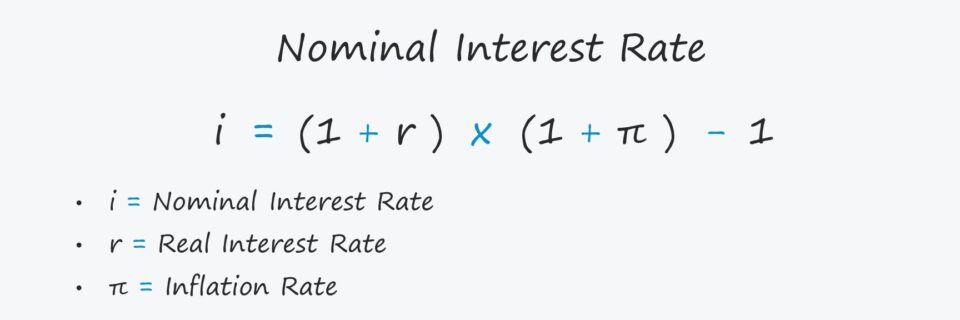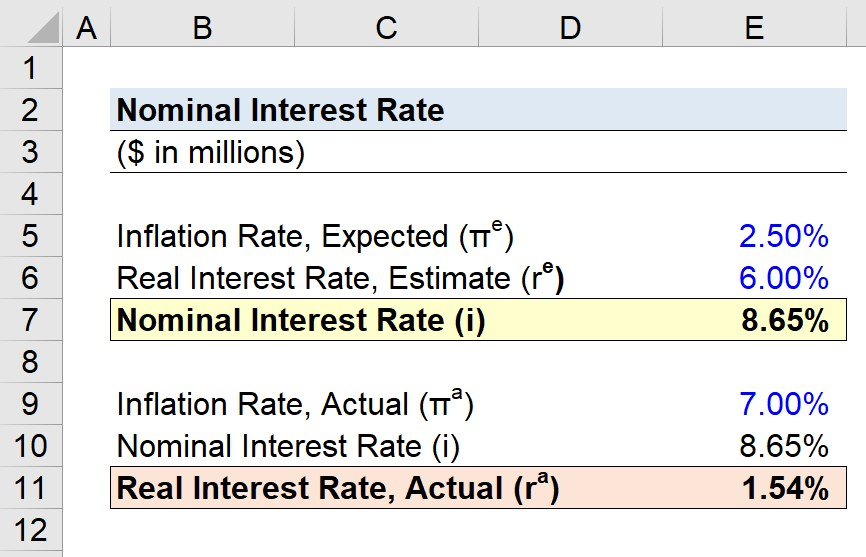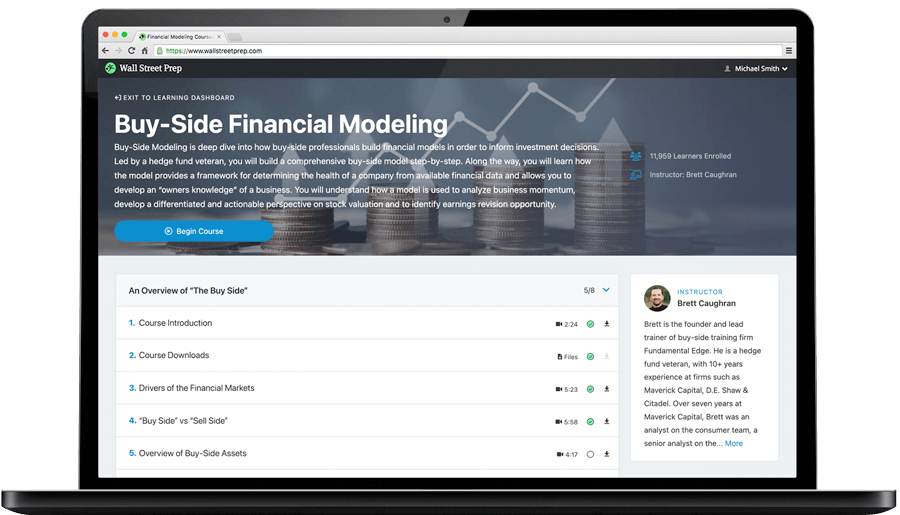Welcome to Wall Street Prep! Use code at checkout for 15% off.# Nominal Interest Rate

Guide to Understanding the Nominal Interest Rate## How to Calculate Nominal Interest Rate (Step-by-Step)

The nominal interest rate is defined as the stated pricing on a financial instrument, which could be related to debt financing such as a loan or a yield-generating investment.

For the everyday consumer, the nominal interest rate is the price quoted on items such as credit cards, mortgages, and savings accounts offered by banks.

The nominal interest rate remains fixed irrespective of the actual inflation rate.

For instance, if new economic data is released that favors the borrower, the interest rate received by the lender is kept the same.

Higher-than-expected inflation can erode the yield earned by a lender because a dollar now is worth less than a dollar on the original date on which the financing arrangement was agreed upon.

In effect, the borrower (i.e. the debtor) tends to benefit from periods of high inflation at the expense of the lender (i.e. the creditor).

Calculating the nominal interest rate requires two inputs:

1. Real Interest Rate → The real interest rate is the actual yield on an investment after adjusting for inflation.
2. Inflation Rate → The rate of inflation refers to the percent increase or decrease in the Consumer Price Index (CPI), which measures the average change over time in the pricing of a market basket consisting of consumer goods and services.

## Nominal Interest Rate Formula

The formula for calculating the nominal interest rate is as follows.

Nominal Interest Rate (i) = [(1 + r) × (1 + π)] 1

Where:

• r = Real Interest Rate
• i = Nominal Interest Rate
• π = Inflation Rate

Note that as a rough approximation, the following equation can be used with reasonable accuracy.

Nominal Interest Rate (i) = r + π

## Nominal vs. Real Interest Rate: What is the Difference?

The interest rate on a financial instrument can be expressed in either nominal or real terms.

• Nominal Interest Rate → The nominal interest rate is the stated interest on a lending agreement, wherein the expected rate of inflation is embedded in the terms of the agreement.
• Real Interest Rate → The real interest rate reflects the cost of borrowing after adjusting for the effects of inflation.

The difference between the nominal interest rate and the real interest rate stems from the effects of inflation. But contrary to a common misconception, it is important to understand that the nominal interest rate does not neglect inflation entirely.

Of course, the nominal interest rate will not explicitly state the anticipated inflation rate, but anticipated inflation is a critical determinant of the interest rate pricing as set by lenders.

On the initial date of the agreement, both parties involves are likely aware of the potential for inflation over time.

The terms are negotiated and structured with that particular risk in mind.

Since the future rate of inflation in a country cannot be determined exactly, the terms are based on projected inflation, which neither party can know with complete certainty.

The differential between the nominal and real interest rate is thus the “excess” over the expected rate of inflation.

Unlike the nominal interest rate, the real interest rate factors inflation into its equation and reflects the actual return earned. Hence, lenders such as commercial or corporate banks pay closer attention to the real interest rate (i.e. estimated return vs. actual return).

## Nominal Interest Rate Calculator — Excel Template

We’ll now move on to a modeling exercise, which you can access by filling out the form below.Submitting...

## Step 1. Lender Loan Agreement Assumptions

Suppose a corporation decided to raise capital in the form of bonds from an institutional lender.

Given the corporation’s credit rating profile and the current market sentiment regarding inflation, the lender must decide the interest rate to charge the borrower.

On the date of the financing arrangement, the expected inflation rate as determined by the lender is 2.50%, and the lender’s minimum target yield (i.e. the real interest rate) is 6.00%.

• Inflation Rate (π), Expected = 2.50%
• Real Rate (r), Estimated = 6.00%

## Step 2. Nominal Interest Rate Calculation Example

Using the assumptions outlined above, we’ll enter those into our formula for calculating the nominal interest rate.

• Nominal Interest Rate (i) = [(1 + 6.00%) × (1 + 2.50%)] −1 = 8.65%

Therefore, given the expected inflation rate of 2.50% and the estimated real rate of 6.00%, the implied nominal rate is 8.65%, which is the institutional lender’s minimum target yield.

## Step 3. Real Interest Rate Analysis (Expected vs. Actual Inflation)

In the final part of our exercise, we’ll assume the actual inflation rate was substantially higher than the anticipated rate of the lender.

The lender had originally expected inflation to be near 2.50% on the date of the financing, but the actual rate of inflation came out to 7.00% instead.

• Inflation Rate (π), Actual = 7.00%

Since the nominal interest rate remains constant, we can rearrange the formula to calculate the real interest rate earned by the lender.

• Real Interest Rate (r), Actual = [(1 + 8.65%) ÷ (1 + 7.00%)] −1 = 1.54%

In closing, the lender missed their target yield by a substantial margin due to the sudden spike in inflation.Step-by-Step Online Course

### Learn Buy-Side (Hedge Fund) Modeling

Led by a former hedge fund PM (Maverick, Citadel, DE Shaw, Schonfeld), this program begins where financial modeling training ends — with a deep-dive into how buy-side analysts build financial models to make key investment decisions.